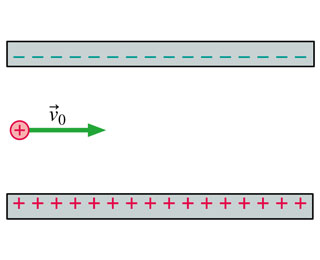# Problem: A parallel-plate capacitor is charged to 5000 V. A proton is fired into the center of the capacitor at a speed of 3.7105m/s as shown in (Figure 1) . The proton is deflected while inside the capacitor, and the plates are long enough that the proton will hit one of them before emerging from the far side of the capacitor. What is the impact speed of the proton?

###### FREE Expert Solution

Consider the kinematic equation:

From Newton's second law,

$\overline{){\mathbf{F}}{\mathbf{=}}{\mathbf{m}}{\mathbf{a}}}$, a = F/m

F = qE, but E = V/d

Therefore, a = Vq/md

Δy = d/2

99% (286 ratings)###### Problem Details

A parallel-plate capacitor is charged to 5000 V. A proton is fired into the center of the capacitor at a speed of 3.7105m/s as shown in (Figure 1) . The proton is deflected while inside the capacitor, and the plates are long enough that the proton will hit one of them before emerging from the far side of the capacitor.What is the impact speed of the proton?